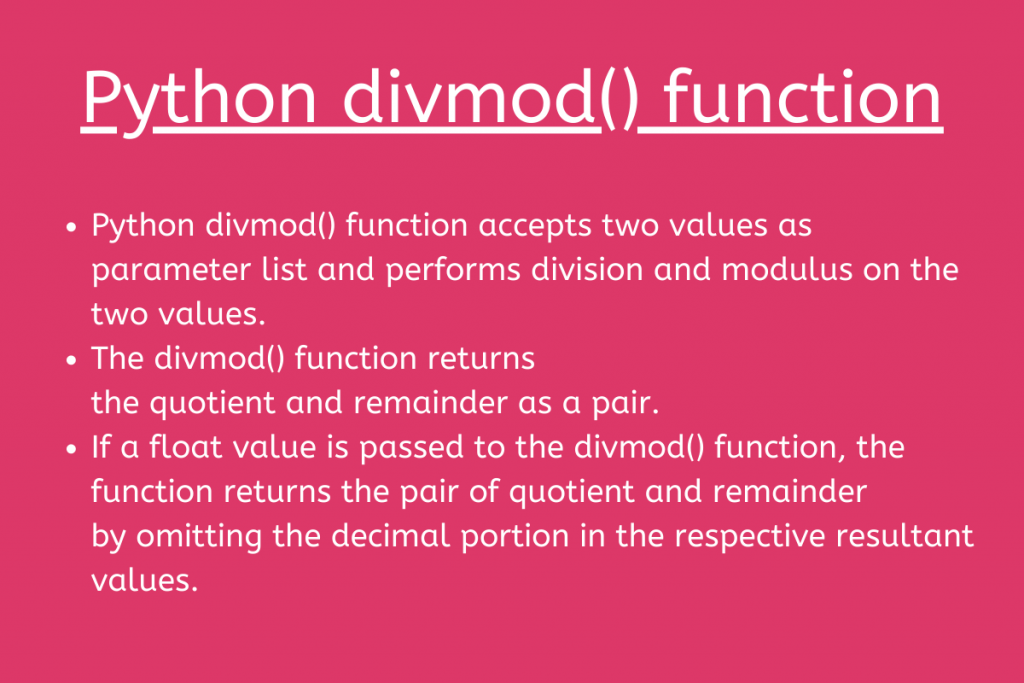# How to use the Python divmod() functionIn this article, we will be unveiling the working and use of Python divmod() function in detail.

## 1. Python divmod() function

Python has an in-built divmod() function which performs division and modulus operation on the two input values.

The `divmod()` function takes two values as arguments and performs division i.e. value1/value2 and modulus operation i.e. value1%value2, and returns the quotient and remainder as a pair.

Syntax:

```divmod(value1, value2)
```
• value1 is treated as nominator
• value2 is treated as denominator

Example 1:

```x= 10
y= 2

res_div = divmod(x,y)
print(res_div)
```

In the above example, we have passed 10 and 2 to the divmod() function. Further, the divmod() function performs division i.e. 10/2 and modulus operation i.e. 10%2 and returns the quotient and the remainder out of it.

Output:

```(5, 0)
```

Example 2:

```x= int(input())
y= int(input())

res_div = divmod(x,y)
print(res_div)
```

Output:

```(2, 0)
```

Example 3:

```x= 10
y= 3

res_div = divmod(x,y)
print(res_div)
```

Output:

```(3, 1)
```

### Python divmod() function with float values

When the divmod() function encounters float values as arguments, the function calculates the quotient and the remainder in a similar manner as shown above.

But, when a float value is passed as an argument to the divmod() function, it returns the quotient value considering only the whole portion of the value obtained i.e. it omits the decimal portion.

Example:

```x= 10.5
y= 5

res_div = divmod(x,y)
print(res_div)
```

As seen above, divmod(10.5, 5) will return (2.0, 0.5) because when float values are encountered, it omits the decimal portion in the result i.e. 10.5/5 would be 2.0 instead of 2.1. Thus, omitting the decimal portion.

Output:

```(2.0, 0.5)
```

Example 2:

```x= 10
y= 2.4

res_div = divmod(x,y)
print(res_div)
```

Output:

```(4.0, 0.40000000000000036)
```

### Python divmod() function- Errors and Exceptions

1. If the value of the first argument passed to the divmod() function is zero(0), then the function returns a pair as (0, 0).

Example 1:

```x= 0
y= 3

res_div = divmod(x,y)
print(res_div)
```

Output:

```(0, 0)
```

2. If the value of the second argument passed to the divmod() function seems to be zero(0), then the function returns a ZeroDivisionError i.e. divide by zero error.

Example 2:

```x= 5
y= 0

res_div = divmod(x,y)
print(res_div)
```

Output:

```Traceback (most recent call last):
File "main.py", line 4, in <module>
res_div = divmod(x,y)
ZeroDivisionError: integer division or modulo by zero
```

3. If the divmod() function encounters a complex number as an argument, it raises the TypeError exception

Example 3:

```inp1 =10 + 5J
inp2 = 4
res_div = divmod(inp1, inp2)
print(res_div)
```

Output:

```Traceback (most recent call last):
File "main.py", line 4, in <module>
res_div = divmod(inp1, inp2)
TypeError: can't take floor or mod of complex number.
```

## Summary

• Python divmod() function accepts two values as a parameter list and performs division and modulus on the two values.
• The divmod() function returns the quotient and remainder as a pair.
• If a float value is passed to the divmod() function, the function returns the pair of quotient and remainder by omitting the decimal portion in the respective resultant values.
• A ZeroDivisionError is raised if the second argument passed to the divmod() function is zero(0).
• The function raises a TypeError exception, if a complex number is passed as an argument to it.

## Conclusion

Thus, in this article, we have understood the working of Python divmod() function.

## References

• Python divmod() – JournalDev